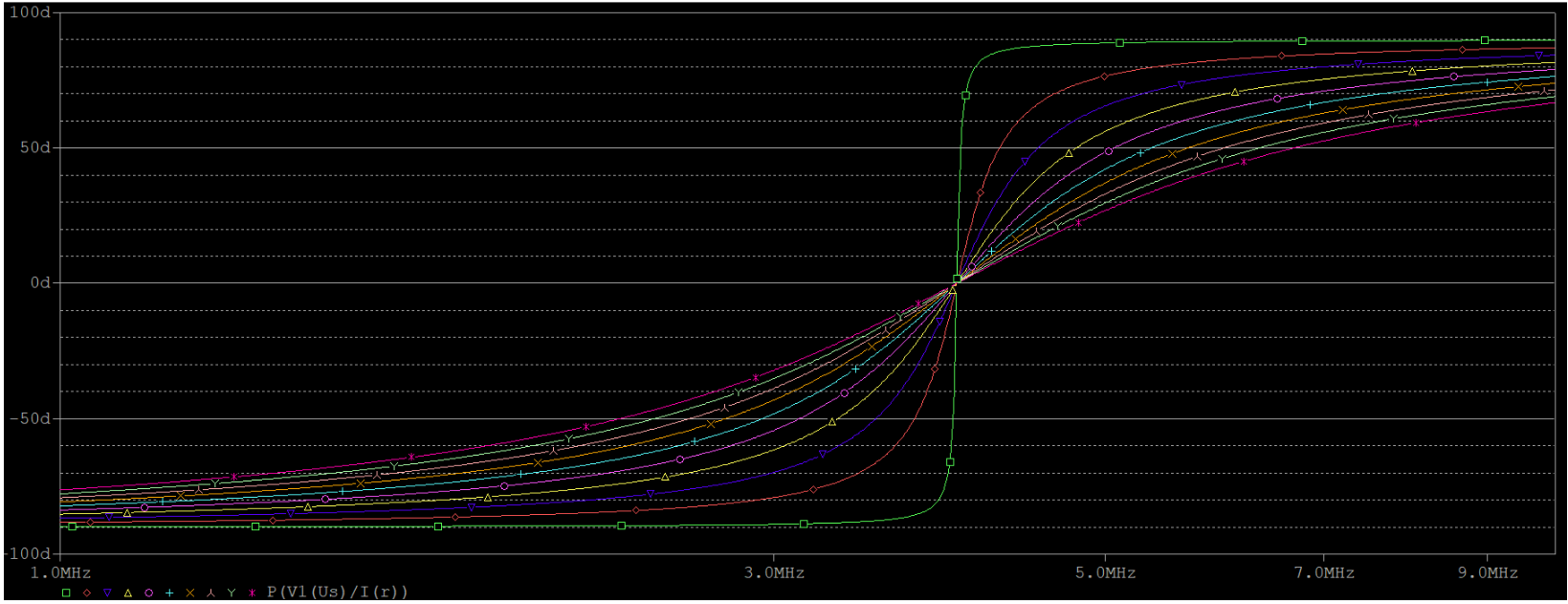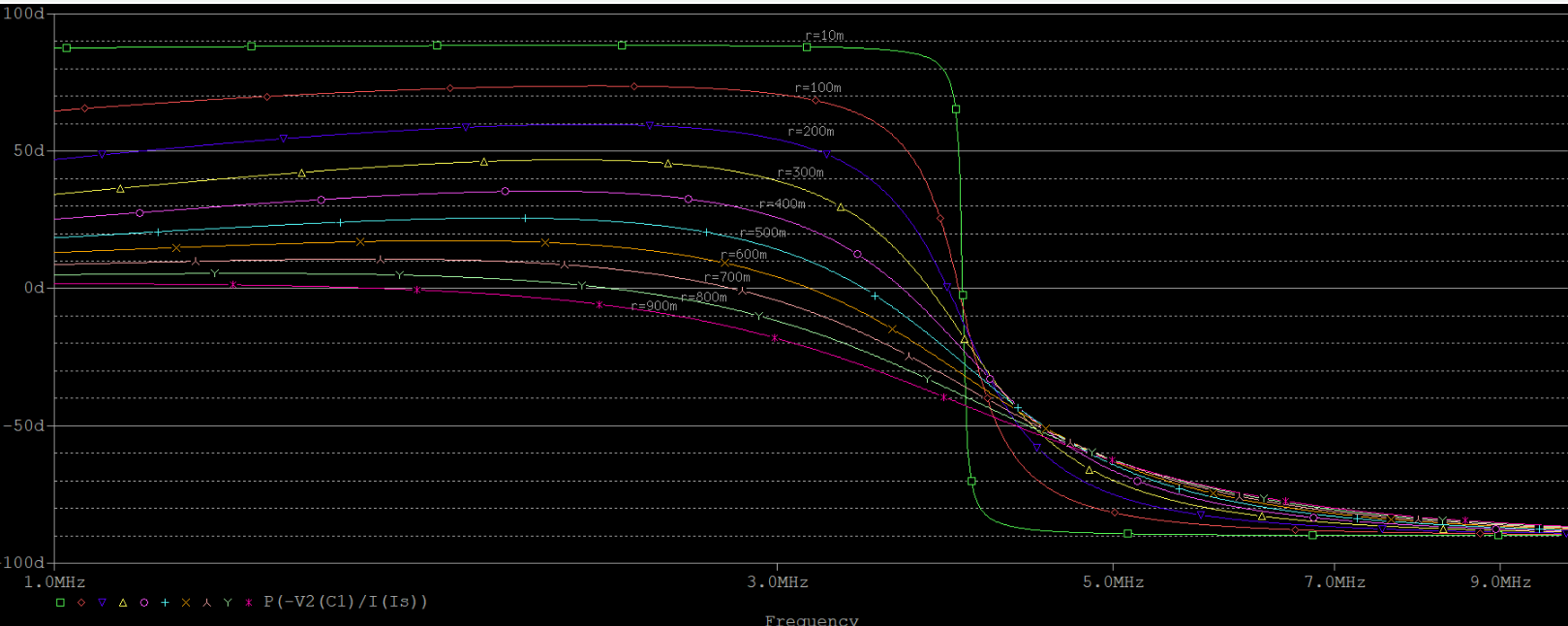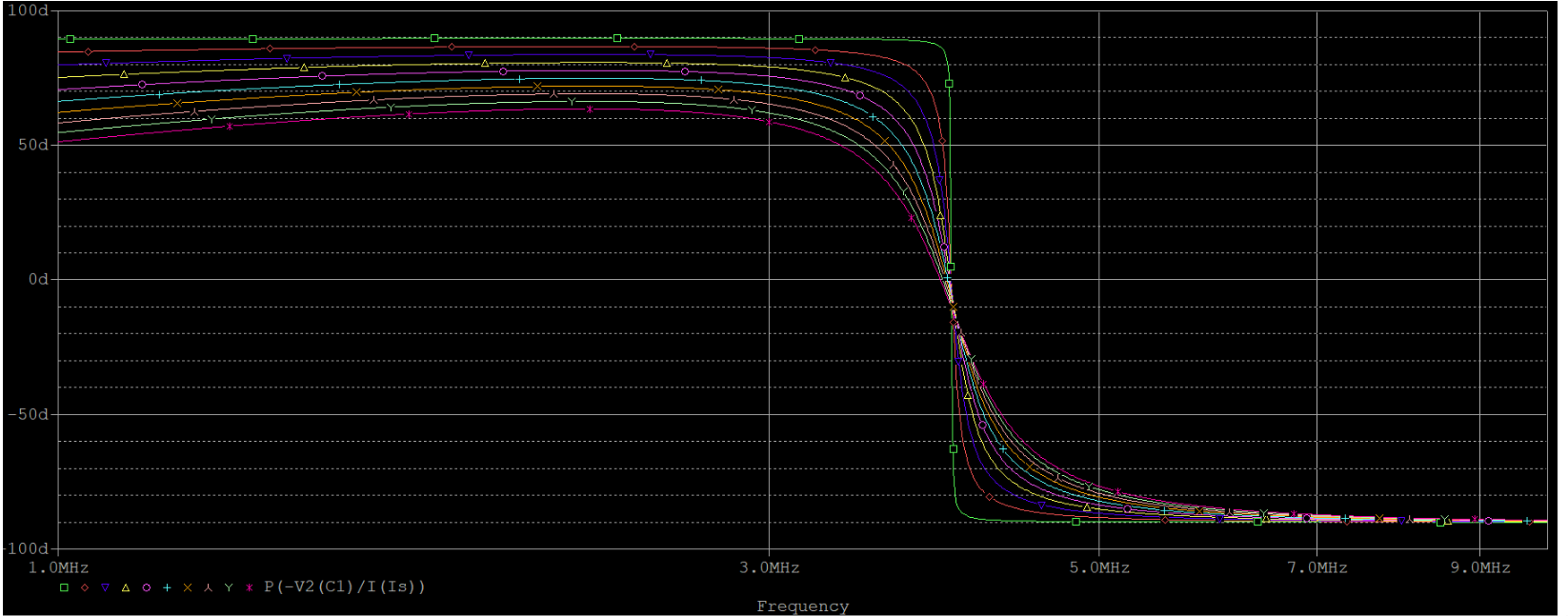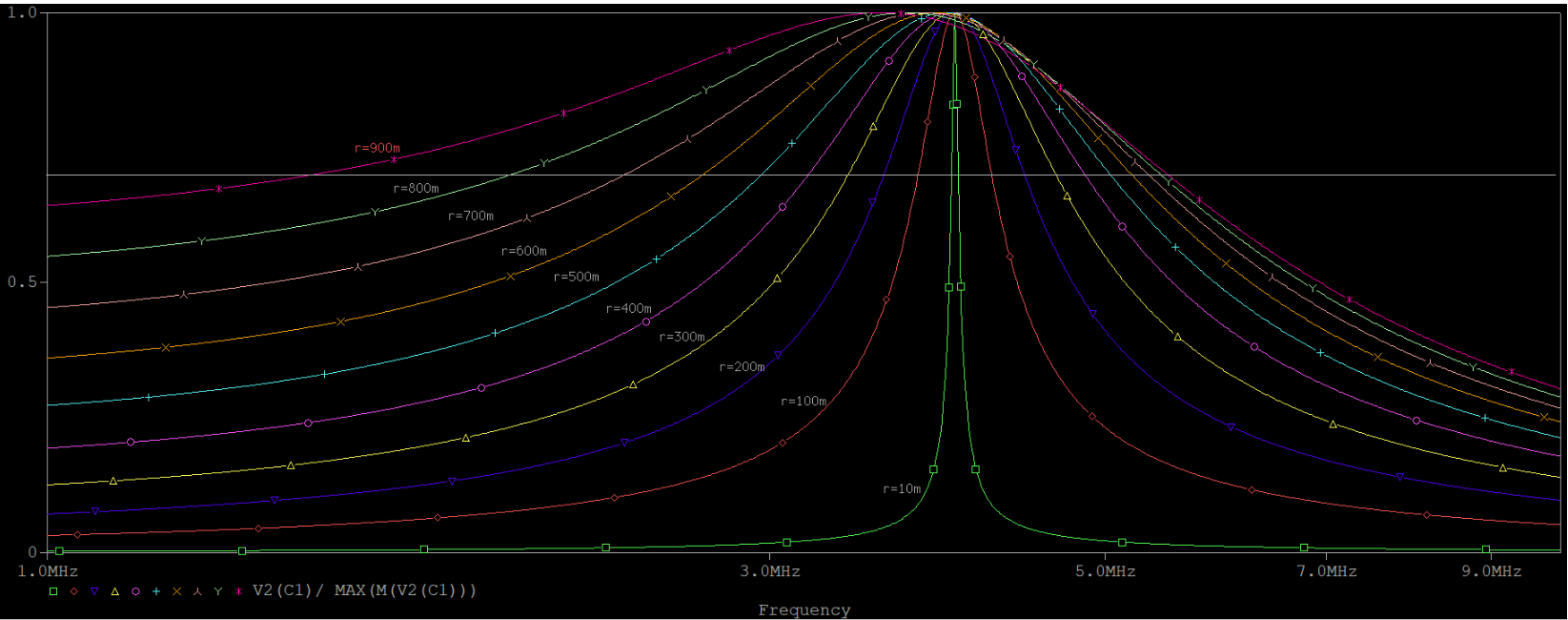# 一阶LC电路的仿真设计案例

KenSporger 发表于 2020-08-10 16:56:10 收藏 已收藏###### 400万+工程师在用# 一阶LC电路的仿真设计案例

KenSporger 发表于 2020-08-10 16:56:10
+加入圈子

LC谐振电路具有选频功能，广泛用于各种通信电路。本文就一阶LC电路进行仿真，以此来进一步加深对电路特性的理解和记忆。

## 谐振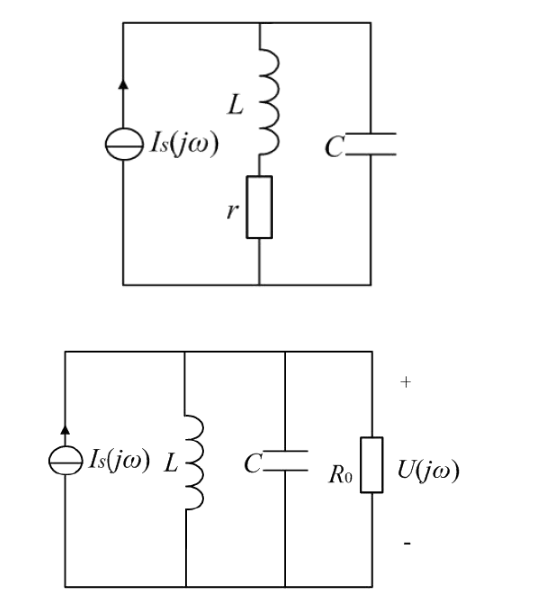1、谐振条件$\omega C = \frac{1}{\omega L}$，满足该条件的谐振角频率$\omega_0 = \frac{1}{\sqrt{L C}}$，谐振频率$f_0=\frac {\omega_0} {2 \pi}$；

1、等效电阻$R（\omega）=\frac{（\omega L）^2}{r}$；

2、发生谐振时，$R（\omega）$记为谐振电阻$R_0$，$R_0=\frac{（\omega_0 L）^2}{r}$；

3、品质因数$Q_0=\frac{R_0}{\omega_0 L}=R_0 \omega_0 C$；

4、通频带$B_0.7=\frac{f_0}{Q_0}$；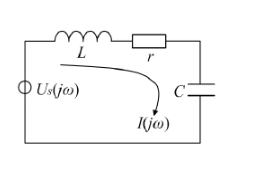1、谐振条件为$\omega L = \frac{1}{\omega C}$，谐振角频率不变，仍然是$\omega_0 = \frac{1}{\sqrt{L C}}$，谐振频率$f_0=\frac {\omega_0} {2 \pi}$。

2、因为电阻r直接作为了阻抗的实部，因此谐振电阻$R_0=r$。

3、品质因数是并联的倒数，$Q_0=\frac{\omega_0 L}{R_0}=\frac{1}{R_0 \omega_0 C}$。

4、通频带$B_0.7=\frac{f_0}{Q_0}$；

## 谐振电路仿真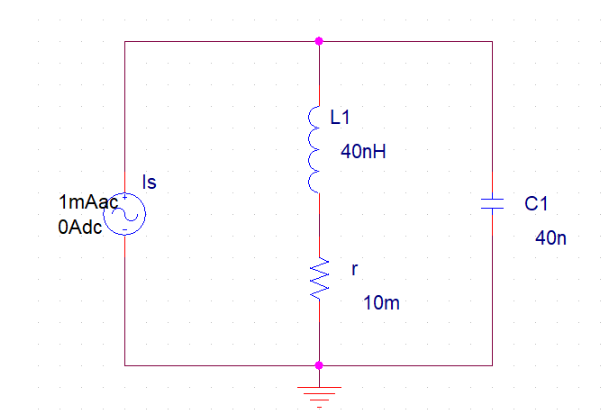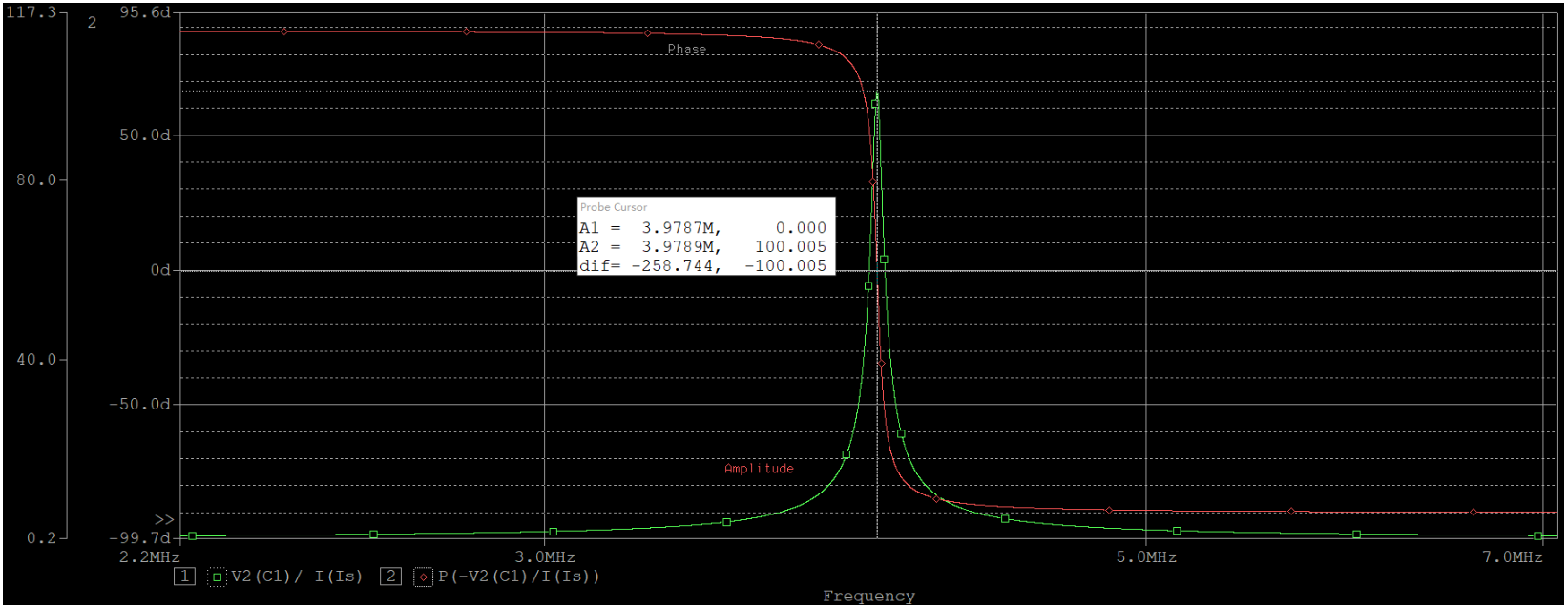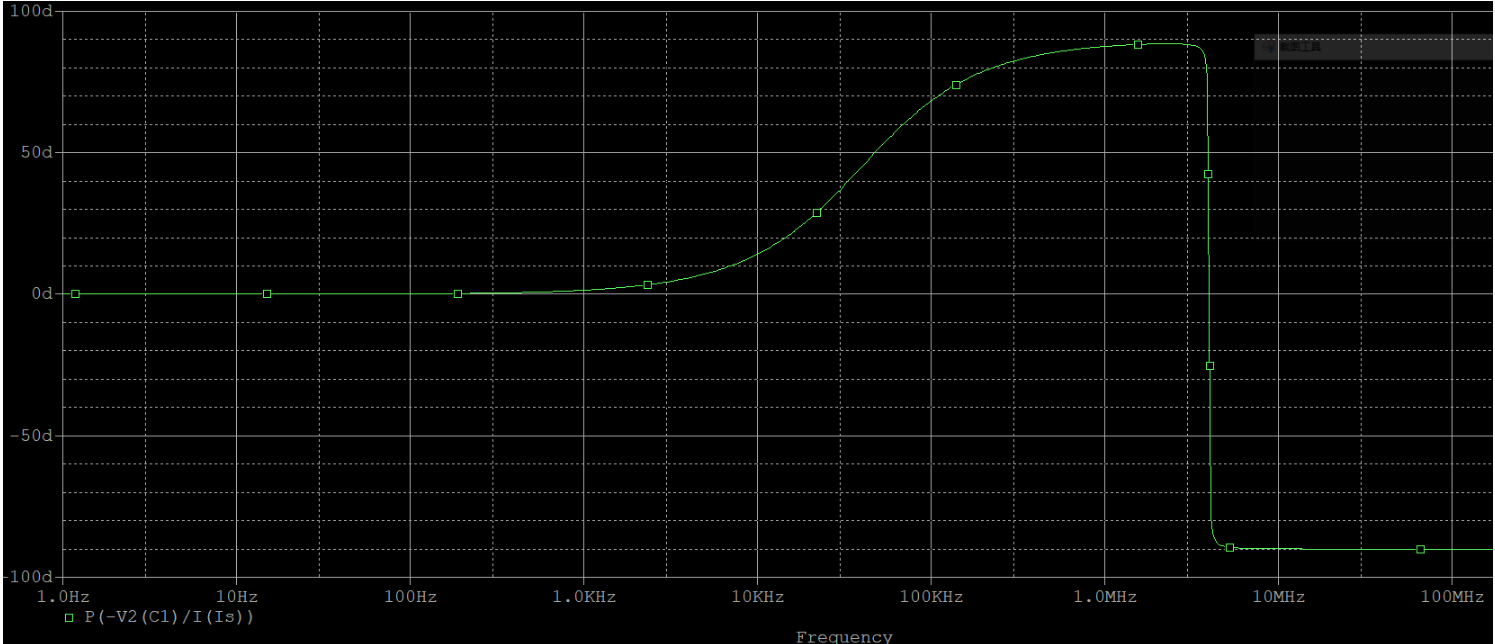$Y（j\omega） = j\omega C + \frac{1}{j\omega L + r}=\frac{r}{r^2 + （\omega L）^2}+j［\omega C - \frac{\omega L}{r^2 + （\omega L）^2}］$

$When \; \omega L 《《 r ，Y（j\omega） = \frac{1}{r}+j［\omega C - \frac{\omega L}{r^2}］$

$\Theta （j\omega） = \arctan \omega （rC-\frac{L}{r}）$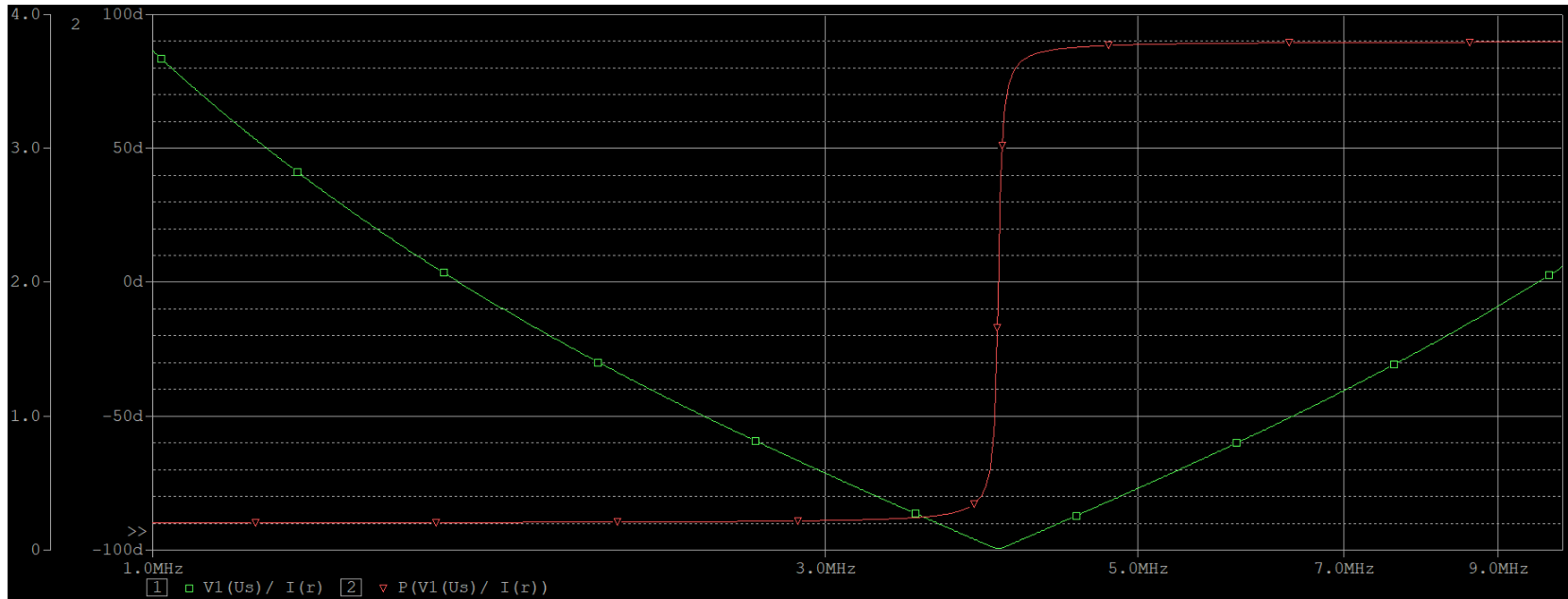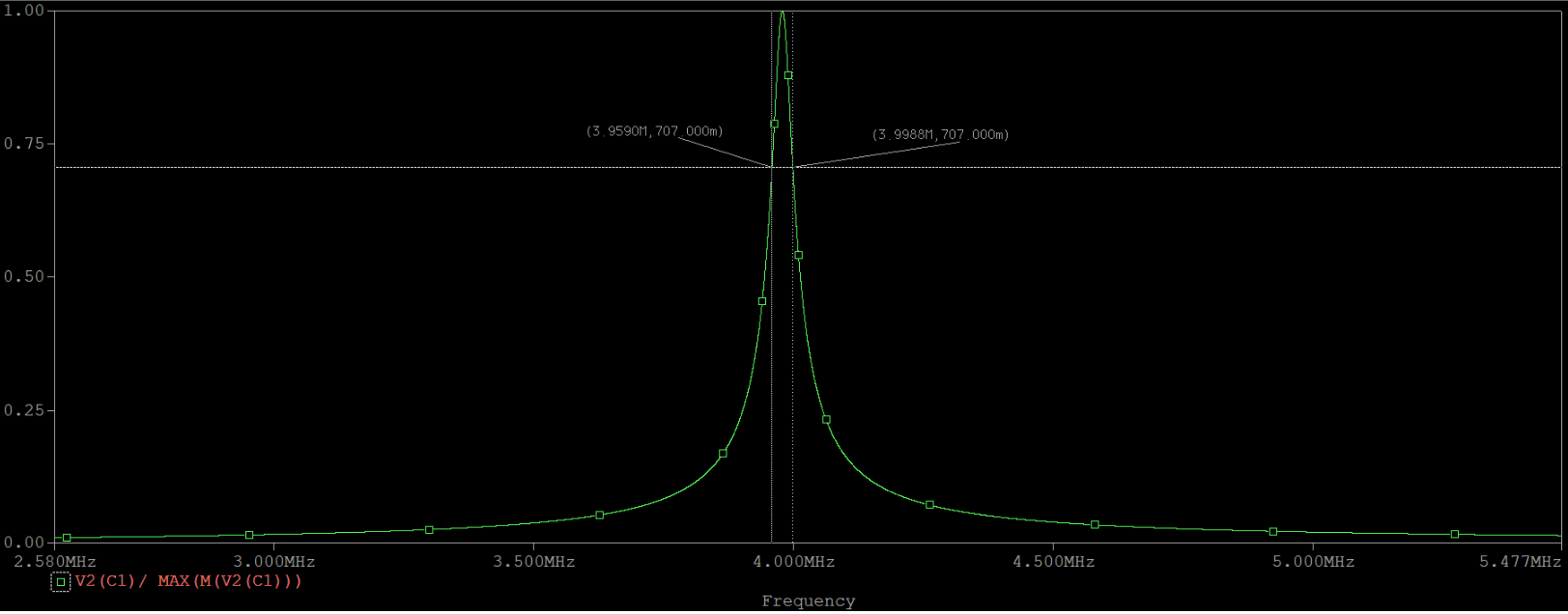## 谐振电路设计